Free Printable Worksheets Covering Addition and Subtraction with Whole Numbers and Fractions

Fraction Subtraction with Same Denominators (Set 2)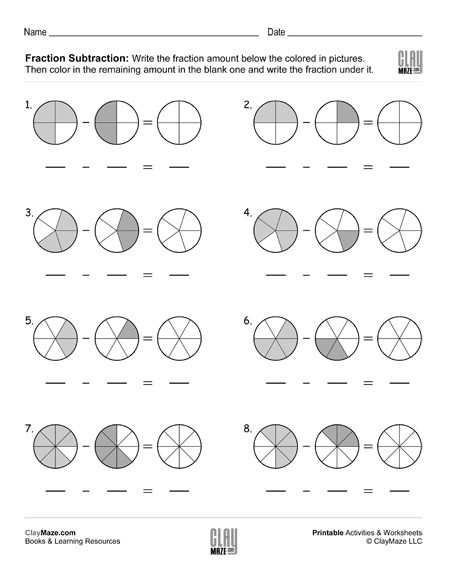Fraction subtraction with common denominators – write the fractions, then subtract them using the pictures and color in the correct amount....

Fraction Subtraction with Same Denominators (Set 3)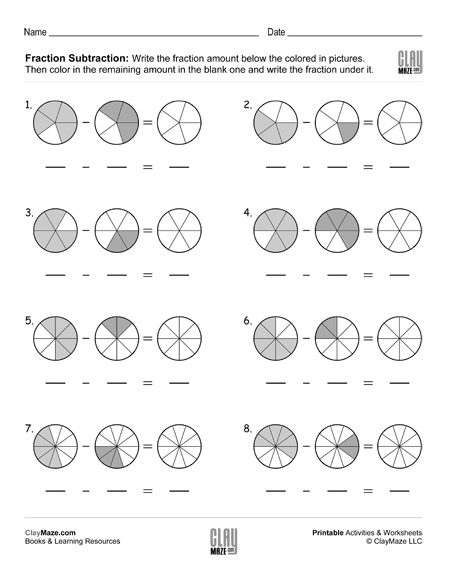Subtracting fractions free, printable worksheet – Write the fractions, subtract them and color the remaining amount....

Fraction Subtraction with Same Denominators (Set 4)Fraction subtraction with common denominators worksheet – Write the correct fraction amount under each picture. Subtract the fractions and color the fractional amount in the blank picture....

Fraction Addition and Simplifying with Coloring (Set 1)Add the fractions and color the correct amount. Simplify the sum....

Fraction Addition and Simplifying with Coloring (Set 2)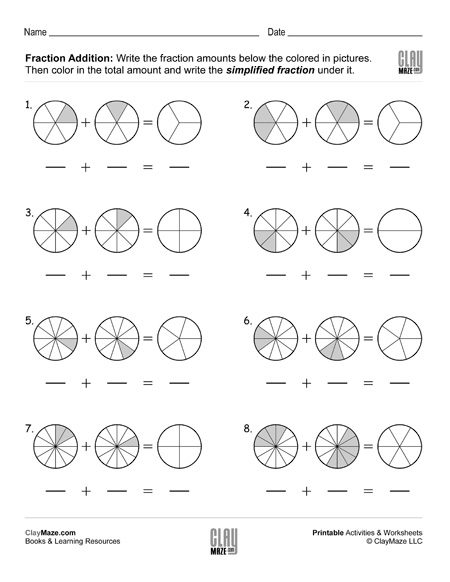Add the fractions and color the correct amount. Simplify the sum....

Subtraction Facts Practice Worksheet (Set 1)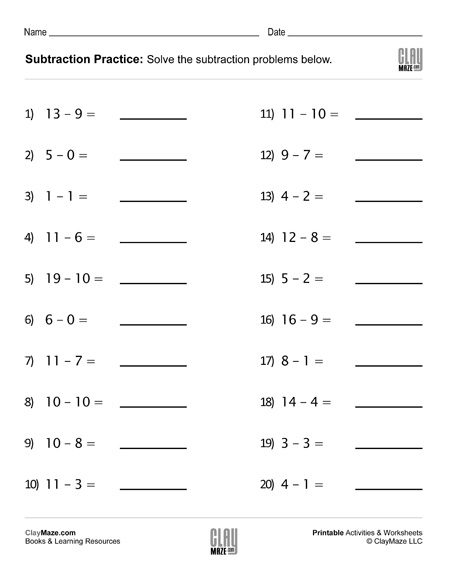Help your child learn and remember the subtraction facts with our subtraction facts worksheets – 20 problems....

Subtraction Facts Practice Worksheet (Set 2)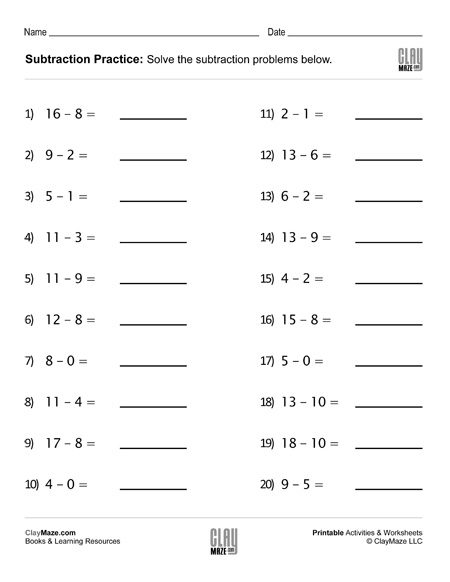Practice the subtraction facts with our subtraction facts worksheets – 20 problems....

Subtraction Facts Practice Worksheet (Set 3)Practice the subtraction facts with our subtraction drills – 20 problems....

Subtraction Facts Practice Worksheet (Set 4)Free worksheet to help learn the subtraction facts – 20 problems....

Addition Facts Practice Worksheet (Set 1)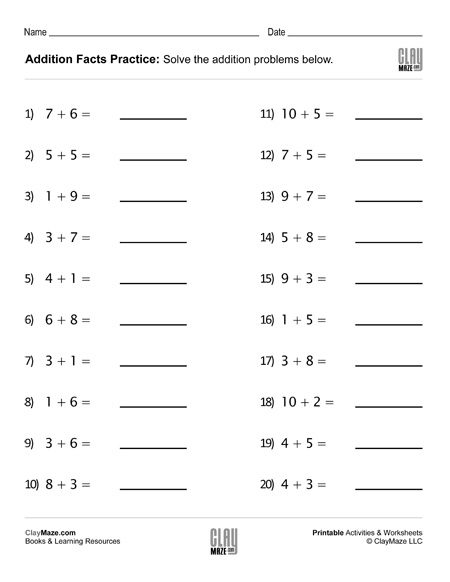Addition Facts Practice Worksheet (Set 2)Practice for addition facts. – 20 problems...

Addition Facts Practice Worksheet (Set 3)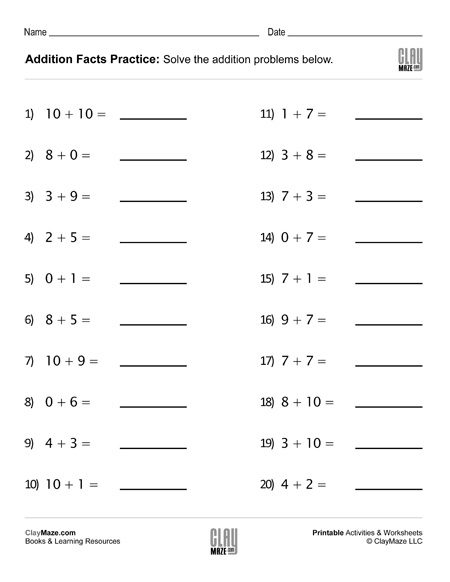Free addition facts worksheet. – 20 problems...

Addition Facts Practice Worksheet (Set 4)Practice the addition facts with this worksheet – 20 problems....

Find the Missing Addends Worksheet (Set 1)Free Math Worksheet – Find the Missing Addends – 20 problems...

Find the Missing Addends Worksheet (Set 2)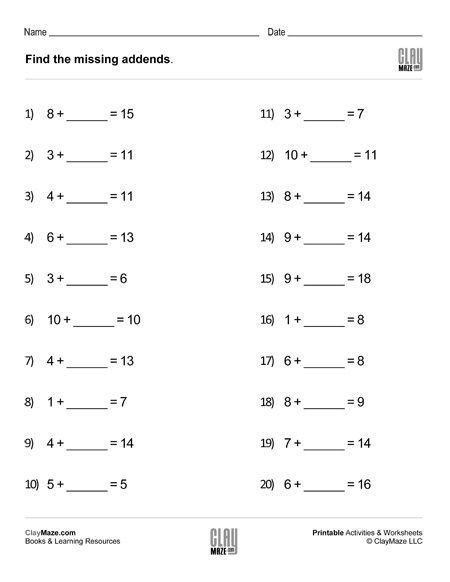Worksheet for addition and subtraction practice. – 20 problems...